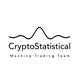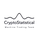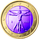# Linear Momentum and Performance Indicators

282 vizualizações
282
This a porting to Trading View of the 12 new indicators introduced in IFTA Journal (January Edition) by Akram El Sherbini, MFTA, CFTe, CETA.

Indicators are available in "Linear Momentum and Performance Indicators" at page four.

IFTA Journal is available below:
http://ifta.org/public/files/journal/d_i...

Indicators implemented herein:
• Linear Force Index: The linear force index LFI measures the force of buyers and sellers during rallies and declines, respectively. It combines two important pieces of market information—the price acceleration
and volumes.
• Pressure Index: The pressure index PRI measures the buying and selling pressure over a certain range within a time interval by moving around its zero line. The index indicates a rise in buying pressure when it crosses above the zero line and a rise in selling pressure
when it crosses below the zero line level. The buying and selling force moves the last price during the session to form a range with low and high boundaries.
• Strength Index Index: The strength index SI is a leading indicator to the pressure index. It measures the ability of buyers to resist sellers and vice versa. SI of today is the ratio of the latest pressure index value to the strain of today.
• Power Index: It measures the buying and selling power within a time interval by moving around its zero line.
• Intensity Index: The intensity index II measures the buying and selling intensity within a time interval by moving around its zero line.
• Dynamic Strength Index: The sole purpose of the dynamic strength index DSI and the integral dynamic strength index IDSI is to lead their intensity indicator peers.
• Integral Force Index
• Integral Pressure Index
• Integral Strength Index
• Integral Power Index
• Integral Intensity Index
• Integral Dynamic Strength Index

The following example shows a trade following the signal while several indicators are crossing the zero line:

Integral performance indicators have a fewer number of trades than the performance indicators. This result is normal, as the integral indicators are less sensitive than their peers. Moreover, the power, intensity, and dynamic strength are less sensitive than the force, pressure, and strength indicators. The same applies for their integrals. Therefore, the integrals of power, intensity, and dynamic strength indicators are more inclined to be medium-term indicators.

As the paper is suggesting "the linear momentum and the new performance indicators should make a significant change in categorizing several indicators in technical analysis ."

Technical indicators are using biased mathematical implementations. For example Momentum Index is in reality a velocity indicator, Force index a Momentum indicator and so on. From a Physical perspective correct momentum, force, velocity etc. needs to be corrected and re-categorized.
The author also gives important insights in how these indicators can be used "simultaneously to identify price turning points and filter irrelevant divergences."

"This paper will attempt to adjust the price momentum and force concepts introduced by Welles Wilder and Alexander Elder, respectively. By introducing the concept of linear momentum, new indicators will emerge to dissect the market performance into six main elements: market’s force, pressure, strength, power, intensity, and dynamic strength. This will lead to a deeper insight about market action. The leading performance indicators can be used simultaneously to identify price turning points and filter irrelevant divergences. The linear momentum and the new performance indicators should make a significant change in categorizing several indicators in technical analysis ."

Suggestions and feedbacks are welcome

Hope you enjoy this,
CryptoStatistical
May 31
Notas de Lançamento: Note from Akram El Sherbini (original author of the indicators)
From page 13 : for errorneous data or one trade where H=L a fix is to add a negligible fraction to the range (H-L+0.0001)

To fix this special case of division by zero RI=0.0001 has been added to the range
-= Visit us: CryptoStatistical.com - Machine Trading for the Crypto MarketsYou can contact the original Author here in Tradingview @Akram01
RespostaNicely done! I noticed an issue where IntegralStrength was not plotting. Adding extra parantheses to line 70 fixed the issue.

70 IntegralStrengthToday= Momentum/(SF*(Range-RealBody))
Respostaallanster
@allanster, from the Author

Hi